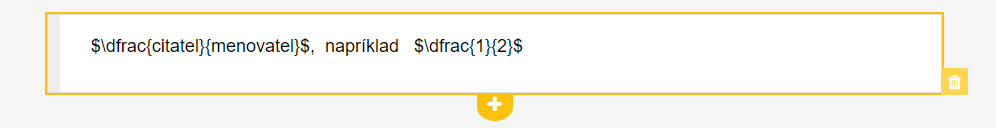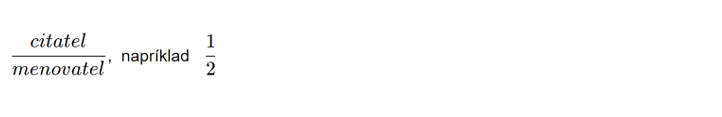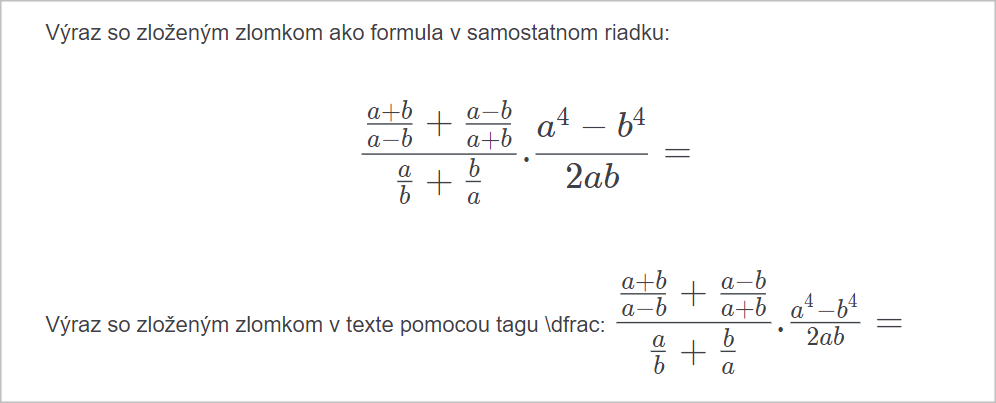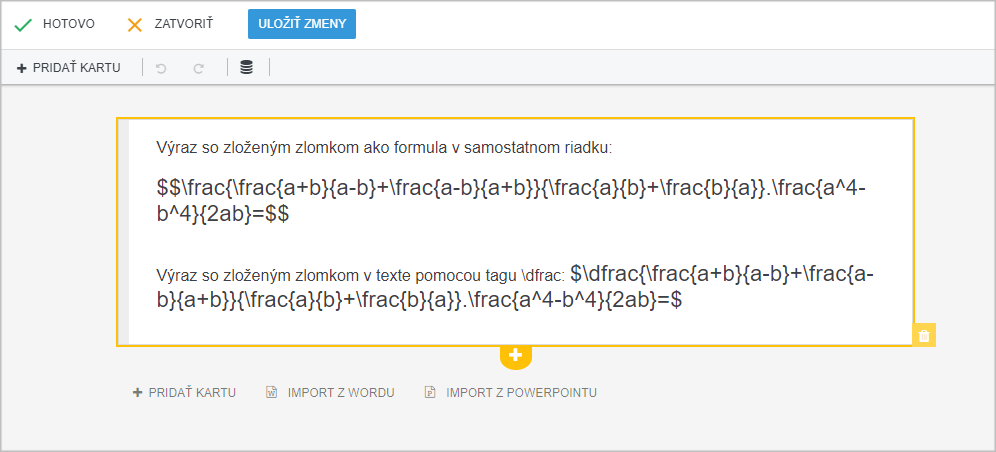EN# How to enter a fraction in preparations?

The teacher can write the fractions in the so-called mathematical mode using LaTeX syntax as follows:

$\frac{numerator}{denominator}$, e.g. $\frac{1}{2}$Result after saving the card:If you use complex fractions , for better readability we recommend either using the formula, ie. an expression enclosed in two dollars $$...$$ or if you need to have a complex fraction in a line, use the command \dfrac{numerator}{denominator} . The \dfrac command renders a larger fraction.This is what these terms look like: